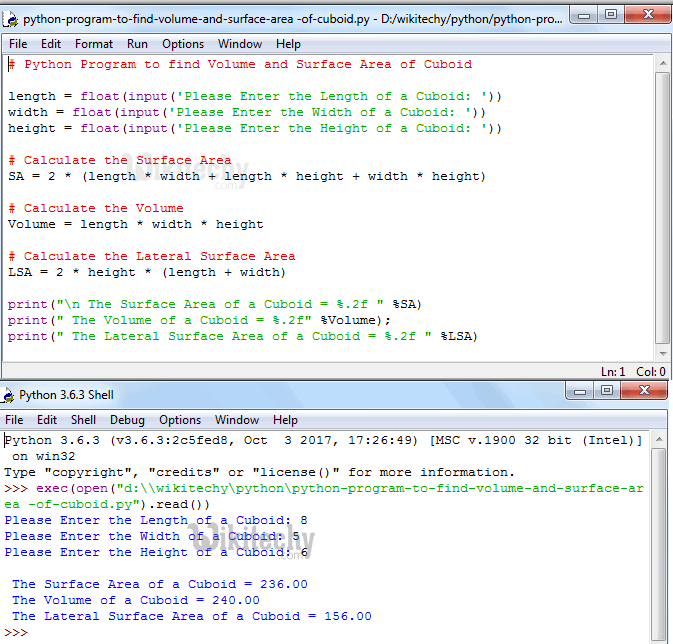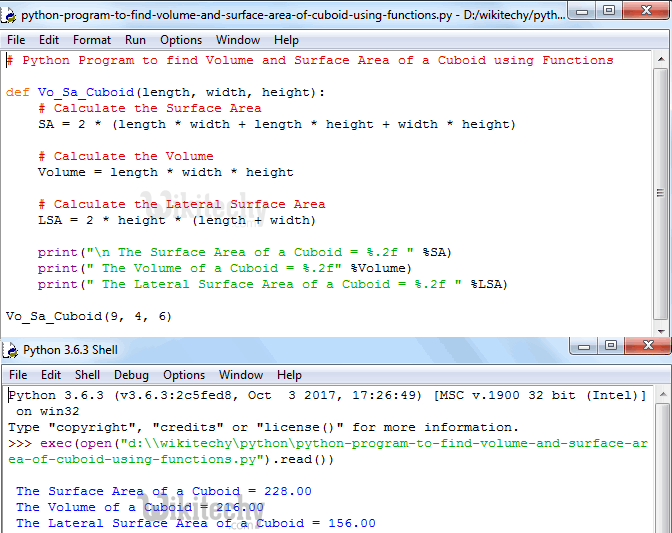# python tutorial - Python Program to find Volume and Surface Area of Cuboid - learn python - python programming

• To write Python Program to find Volume and Surface Area of Cuboid with example.
• Before we step into the program, Let see the definitions and formulas behind Surface area of a Cuboid, Area of Top & Bottom Surfaces, Lateral Surface Area and Volume of a CuboidLearn Python - Python tutorial - Python Program to find Volume and Surface Area of Cuboid - Python examples - Python programs

## Cuboid

• Cuboid is a 3D object made up of 6 Rectangles. All the opposite faces (i.e Top and Bottom) are equal.

## Surface Area of a Cuboid

• The Total Surface Area of a Cuboid is the sum of all the 6 rectangles areas present in the Cuboid.
• If we know the length, width and height of the Cuboid then we can calculate the Total Surface Area using the formula:
• The Total Surface Area of a Cuboid is the sum of all the 6 faces. So, we have to add all these area to calculate the final Surface Area

## Volume of a Cuboid

• The amount of space inside the Cuboid is called as Volume.
• If we know the length, width and height of the Cuboid then we can calculate the Volume using the formula:

## Python Program to find Volume and Surface Area of Cuboid

• This Python program allows user to enter the length, width and height of a Cuboid.
• Using these values, compiler will calculate the Surface Area of a Cuboid, Volume of a Cuboid and Lateral Surface Area of a Cuboid as per the formulas.

## Analysis

• Below statements will ask the user to enter length, width and height values and it will assign the user input values to respected variables.
• Such as first value will be assigned to length, second value to width and third value will be assigned to height
• Next, We are calculating Volume, Surface Area and Lateral Surface Area of a Cuboid using their respective Formulas:
• Following print statements will help us to print the Volume and Surface area of a Cuboid

## OutputLearn Python - Python tutorial - Python Program to find Volume and Surface Area of Cuboid - Python examples - Python programs

## Analysis

• In the above Example, We inserted Values Length = 8, Width = 5 and Height = 6

The Volume of a Cuboid for the Given Measures are:
Volume of a Cuboid = lbh = l * w * h
Volume of a Cuboid = length * width * height
Volume of a Cuboid = 8 * 5 * 6
Volume of a Cuboid = 240
The Volume of a Cuboid is 240

The Total Surface Area of a Cuboid for the Given Measures are:
Total Surface Area of a Cuboid = 2lw + 2lh + 2wh
Total Surface Area of a Cuboid = 2 (lw + lh +wh)
Total Surface Area of a Cuboid = 2*(length * width + length * height + width * height)
Total Surface Area of a Cuboid = 2 * ( (8 * 5) + (8 * 6) + (5 * 6) )
Total Surface Area of a Cuboid = 2 * (40 + 48 + 30)
Total Surface Area of a Cuboid = 2 * 118
Total Surface Area of a Cuboid = 236
The Total Surface Area of a Cuboid is 236

## Python Program to find Volume and Surface Area of Cuboid using functions

• This program allows user to enter the length, width and height values.
• We will pass those values to the function argument and then it will calculate the Surface Area and Volume of a Cuboid as per the formula.

## Analysis:

• First, We defined the function with three arguments using def keyword.
• It means, User will enter the length, width and height values of a Cuboid.
• This program will calculate the Surface Area and Volume of Cuboid as we explained in first example

## OutputLearn Python - Python tutorial - Python Program to find Volume and Surface Area of Cuboid using functions - Python examples - Python programs

NOTE: We can call the function with arguments in .py file directly or else we can call it from the python shell. Please don’t forget the function arguments